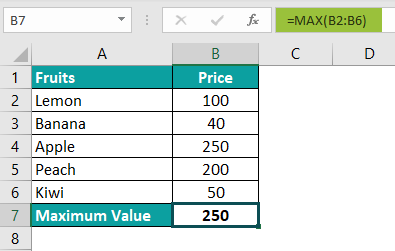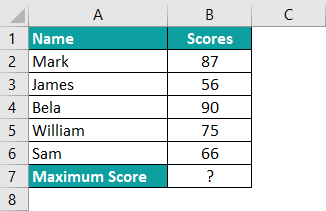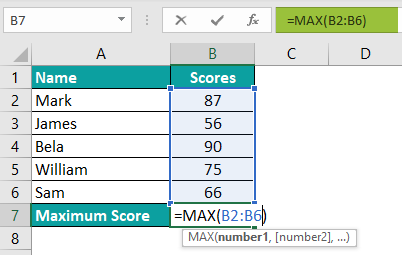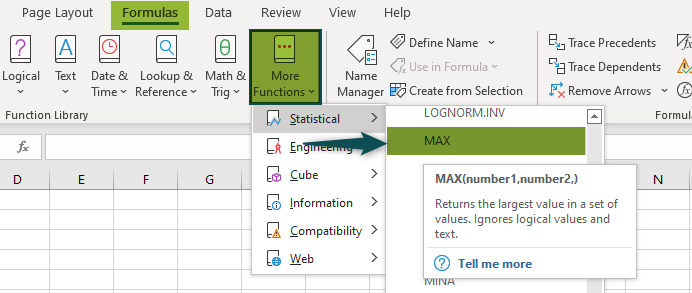# MAX Excel FunctionArticle byExcelMojo Team## What Is MAX Function In Excel?

The MAX function of Excel finds the maximum (or largest) value from a list of numeric values. It is a Statistical function of Excel, which returns only a numeric value as the output. The MAX function is available in almost all versions of Excel.

For example, in the following image, the formula “=MAX(A2:A6)” returns the maximum number of the range A2:A6.

The output of the MAX formula is 67.

###### Key Takeaways
• The MAX function of Excel helps find the maximum value from a range of numeric values. It is a Statistical function of Excel.
• The syntax of the MAX function is “MAX(number1,[number2],…).” The numbers are the values or cell references whose maximum is to be found. Only the first argument is mandatory.
• The MAX function returns 0 if an empty range is supplied or none of the arguments is a number.
• The MAXA function finds the maximum value from a list of numbers, logical values (true and false), and text representations of numbers.
• The MAXIFS function returns the maximum value based on one or more conditions.

### Syntax of the MAX() Function of Excel

The syntax of the MAX function of Excel is shown in the following image:

The MAX function accepts the following arguments:

• Number1: This is the first number, cell reference or range whose maximum is to be found.
• Number2: This is the second number, cell reference or range whose maximum is to be found.

The “number1” argument is mandatory, while the subsequent number arguments are optional. The MAX function can also be supplied named ranges consisting of numbers.

#### MAX Function Notes

The MAX function treats the empty cells, logical values (true and false), and text strings as follows:

• It ignores the cell references of empty cells, logical values, and text strings.
• It counts the logical values that are supplied to it directly. The values “true” and “false” are counted as 1 and 0 respectively.
• It counts the text representations of numbers regardless of whether they are supplied directly (in double quotes) or as cell references. For instance, the formula =MAX(3,4,5,“7”) returns 7.
• It returns the “#VALUE!” error if a text string is supplied to it directly within double quotation marks. For instance, the formula =MAX(9,12,”d”,5) returns the “#VALUE!” error.

Note: The MAX function can handle up to 255 arguments in Excel 2007 and the subsequent versions.

### How to Use the MAX Function in Excel?

The MAX function of Excel can be used in either of the following ways:

A. Access the MAX function from the Excel ribbon

1. Choose an empty cell that will contain the output.
2. Click the Formulas tab of Excel.
3. Expand the “more functions” drop-down from the “function library” group.
4. Select “statistical” and choose “MAX” from the list of functions. A window called “function arguments” appears.
5. Specify the arguments in the “number1” and “number2” boxes.
6. Click “Ok” to find the maximum value.

B. Enter the MAX function in the worksheet manually

1. Select an empty cell for the output.

2. Open the MAX function by typing “=MAX(” in the selected cell. Alternatively, type “=M” and double-click the MAX function shown in the list of suggestions.

3. Supply the arguments to the MAX function.

4. Close the parenthesis and press the “Enter” key.

### Basic Example

The image below shows the names and the prices of fruits (in \$) in columns A and B, respectively. Find the fruit that has the maximum price by using the MAX function of Excel.

The steps to find the maximum price by using the MAX function of Excel are listed as follows:

Step 1: Select cell B7 as this will contain the output.

Step 2: Enter the following formula (without the double quotation marks) in cell B7.

“=MAX(B2:B6)”

To supply the range to the MAX function, select cells B2 to B6. Alternatively, one can type the range B2:B6 in the formula manually.

Step 3: Press the “Enter” key. The output in cell B7 is 250, as shown in the following image. Hence, the maximum number of the range B2:B6 has been found by the MAX function of Excel.

####### Example #1–Using the MAXA Function When the Inputs Are In Different Formats

The MAXA function belongs to the same family as that of the MAX function. The MAXA function finds the maximum value from a list of numbers, logical values (true and false), and text representations of numbers.

The syntax of the MAXA function of Excel is stated as follows:

“=MAXA(value1,[value2],…)”

The arguments can be numbers, cell references, text representations of numbers, or logical values. “Value1” is a mandatory argument, while the subsequent value arguments are optional.

The MAXA function treats the empty cells, logical values (true and false), and text strings as follows:

• It ignores the empty cells.
• It counts the value “true” as 1 and “false” as 0 irrespective of whether the logical values are supplied directly or as cell references.
• It counts the cell references containing text strings as 0. However, the text strings supplied directly within double quotes cause “#VALUE!” errors. For instance, the formula =MAXA(2,4,”p”,9) returns the “#VALUE!” error.
• It counts the text representation of numbers regardless of whether it is supplied directly or as cell references. For instance, the formula =MAXA(-5,-6,”1″,-2) returns 1.

Note: The MAXA function can accept up to 255 arguments in Excel 2007 and the newer versions.

The succeeding image shows some integers, percentages, and decimal numbers in column A. Perform the following tasks in Excel:

• Find the maximum number of the range A2:A7 by using the MAXA function of Excel.
• State whether the blank cell of column A is included or not while arriving at the output.

The steps to find the maximum number by using the MAXA function are listed as follows:

Step 1: Select a blank cell where the output will be displayed. So, select cell B2, which contains a question mark.

Step 2: Enter the following MAXA formula in cell B2.

“=MAXA(A2:A7)”

To enter the range, either select it or type the references manually.

Step 3: Press the “Enter” key. The result is shown in cell B2 of the following image.

Hence, the integer 667 is the largest of all the values of the range A2:A7. The MAXA function has ignored the empty cell A6 while looking for the maximum value in the stated range.

#### Example #2–Using The MAXIFS Function When a Single Condition Is To Be Applied

The MAXIFS function identifies the maximum value from the specified range based on single or multiple criteria (conditions).

The syntax of the MAXIFS function is stated as follows:

“=MAXIFS(max_range,criteria_range1,criteria1,[criteria_range2,criteria2],…)”

The “max_range” is the range in which the maximum value is to be found. The “criteria_range1” is the range to be evaluated against the first criteria (or “criteria1”). “Criteria1” is the first condition to be evaluated.

All these three arguments are mandatory. But, the subsequent range/criteria pairs are optional.

Note: The MAXIFS can accept up to 126 range/criteria pairs. This function was introduced in Excel 2019.

The following image shows the revenues (in \$ in column C) generated in certain months (column B) by certain regions (column A). Find the maximum revenue generated by the Northern region. Use the MAXIFS function of Excel.

The steps to find the maximum revenue generated by the Northern region are listed as follows:

Step 1: Open the MAXIFS function in cell C8. Enter the “max_range” as C2:C7. This is the range whose maximum is to be found.

For supplying the range, either select it or type the references manually.

Step 2: Enter “criteria_range1” as A2:A7. This is the range to be evaluated against the given criterion (North region).

Step 3: Supply the “criteria1” as “North.” This is because the maximum sales of the Northern region need to be found.

Step 4: Press the “Enter” key. The output in cell C8 is 1733. This is shown in the following image.

In the preceding image, the range A2:A7 is evaluated against the condition “North.” Only cells A2 and A5 meet this condition.

Since the maximum has to be found from the range C2:C7, the MAXIFS function considers only the numbers of cells C2 (1580) and C5 (1733) while arriving at the output. This is because only cells C2 and C5 belong to the Northern region. Hence, the MAXIFS has returned the higher of these two values, that is, 1733.

#### Example #3–Using The MAX Function When The Input Is a Blank Cell Reference

The following image shows cell A2 as a blank cell. Supply this single cell reference to the MAX function and observe the outcome.

The steps to supply a blank cell reference to the MAX function are listed as follows:

Step 1:  Select cell B2 for the output.

Step 2: Enter the following MAX formula in cell B2.

“=MAX(A2)”

Step 3: Press the “Enter” key. The output in cell B2 is 0, as shown in the following image.

Therefore, the MAX function returns 0 when a blank cell reference is supplied to it. However, if a range consisting of a mix of numeric values and empty cells is supplied to the MAX function, it returns the largest of the numeric values. The empty cells are ignored in this case.

#### Example #4–Using The MAX Function When The Maximum Value Is To Be Highlighted

The succeeding image shows the revenue generated (in \$ in column B) from certain products (in column A) of an organization. Highlight the maximum revenue from the range B2:B9 by using the MAX function of Excel.

The steps to highlight the maximum revenue by using the MAX function are listed as follows:

Step 1: Select the column in which the maximum value is to be highlighted. We have selected column B. Next, perform the following actions:

• Click the Home tab of Excel.
• Click the “conditional formatting” drop-down from the “styles” group.
• Expand the option “top/bottom rules” and select “top 10 items.”

Step 2: A window called “top 10 Items” opens in Excel, as shown in the following image. Perform the following actions in this window:

• In the box to the left, enter 1. This is because only a single maximum value needs to be highlighted.
• In the box to the right, select the desired color. We have selected “light red fill with dark red text.”
• Click “Ok.”

Step 3: The value 1000 is highlighted in column B, as shown in the following image. So, the revenue generated by cakes is the highest.

### Important Things To Note

The important points related to the MAX function of Excel are listed as follows:

• It returns the maximum of the given numeric values.
• It does not sum the numeric values.
• It ignores the cell references of blank cells, logical values, and text strings. However, the logical values supplied directly are counted by the function. But, the direct text strings return the “#VALUE!” error.
• It can handle up to 255 arguments.

1. Define the MAX function of Excel.

The MAX function returns the maximum value from a list of numeric values. Cell references, range references, or named ranges containing numbers can also be supplied to this function. It is an in-built function that is categorized as a Statistical function of Excel.

The syntax of the MAX function of Excel is stated as follows:
“=MAX(number1,[number2],…)”

Note: For details related to the arguments, refer to the heading “syntax of the MAX() function of Excel” given in this article.

2. Give an example of the MAX function of Excel.

Let us find the maximum score by using the MAX function of Excel. The steps are listed as follows:

Step 1: Select cell B7 for the output.Step 2: Enter the formula “=MAX(B2:B6)” without double quotes in cell B7.Step 3: Press the “Enter” key. The output is 90, as shown in the following image. Hence, the MAX function has found the maximum score.3. Where is the MAX function in Excel?

The steps to access the MAX function in Excel are listed as follows:
a. Click the Formulas tab of Excel.
b. Click the “more functions” drop-down from the “function library” group of this tab.
c. Expand the option “statistical” and choose “MAX.”
d. Enter the arguments in the “function arguments” window.
e. Click “Ok” to find the maximum value.

The selections of steps “b” and “c” are shown in the following image.